Kinetics

# 75 Reaction Mechanisms

### Learning Objectives

By the end of this section, you will be able to:

• Distinguish net reactions from elementary reactions (steps)
• Identify the molecularity of elementary reactions
• Write a balanced chemical equation for a process given its reaction mechanism
• Derive the rate law consistent with a given reaction mechanism

Chemical reactions very often occur in a step-wise fashion, involving two or more distinct reactions taking place in sequence. A balanced equation indicates what is reacting and what is produced, but it reveals no details about how the reaction actually takes place. The reaction mechanism (or reaction path) provides details regarding the precise, step-by-step process by which a reaction occurs.

The decomposition of ozone, for example, appears to follow a mechanism with two steps: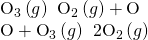Each of the steps in a reaction mechanism is an elementary reaction. These elementary reactions occur precisely as represented in the step equations, and they must sum to yield the balanced chemical equation representing the overall reaction: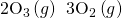Notice that the oxygen atom produced in the first step of this mechanism is consumed in the second step and therefore does not appear as a product in the overall reaction. Species that are produced in one step and consumed in a subsequent step are called intermediates.

While the overall reaction equation for the decomposition of ozone indicates that two molecules of ozone react to give three molecules of oxygen, the mechanism of the reaction does not involve the direct collision and reaction of two ozone molecules. Instead, one O3 decomposes to yield O2 and an oxygen atom, and a second O3 molecule subsequently reacts with the oxygen atom to yield two additional O2 molecules.

Unlike balanced equations representing an overall reaction, the equations for elementary reactions are explicit representations of the chemical change taking place. The reactant(s) in an elementary reaction’s equation undergo only the bond-breaking and/or making events depicted to yield the product(s). For this reason, the rate law for an elementary reaction may be derived directly from the balanced chemical equation describing the reaction. This is not the case for typical chemical reactions, for which rate laws may be reliably determined only via experimentation.

### Unimolecular Elementary Reactions

The molecularity of an elementary reaction is the number of reactant species (atoms, molecules, or ions). For example, a unimolecular reaction involves the reaction of a single reactant species to produce one or more molecules of product: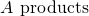The rate law for a unimolecular reaction is first order: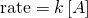A unimolecular reaction may be one of several elementary reactions in a complex mechanism. For example, the reaction: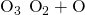illustrates a unimolecular elementary reaction that occurs as one part of a two-step reaction mechanism as described above. However, some unimolecular reactions may be the only step of a single-step reaction mechanism. (In other words, an “overall” reaction may also be an elementary reaction in some cases.) For example, the gas-phase decomposition of cyclobutane, C4H8, to ethylene, C2H4, is represented by the following chemical equation: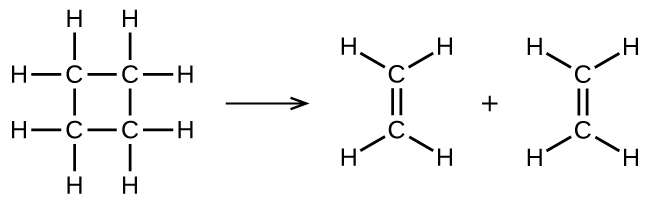This equation represents the overall reaction observed, and it might also represent a legitimate unimolecular elementary reaction. The rate law predicted from this equation, assuming it is an elementary reaction, turns out to be the same as the rate law derived experimentally for the overall reaction, namely, one showing first-order behavior: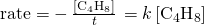This agreement between observed and predicted rate laws is interpreted to mean that the proposed unimolecular, single-step process is a reasonable mechanism for the butadiene reaction.

### Bimolecular Elementary Reactions

A bimolecular reaction involves two reactant species, for example: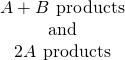For the first type, in which the two reactant molecules are different, the rate law is first-order in A and first order in B (second-order overall):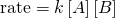For the second type, in which two identical molecules collide and react, the rate law is second order in A: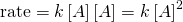Some chemical reactions occur by mechanisms that consist of a single bimolecular elementary reaction. One example is the reaction of nitrogen dioxide with carbon monoxide: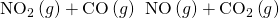(see (Figure))

The probable mechanism for the reaction between NO2 and CO to yield NO and CO2.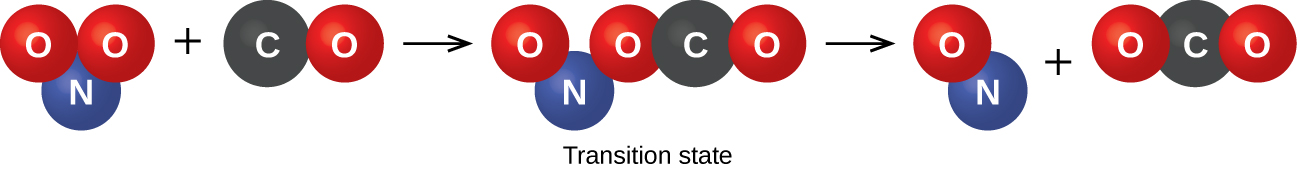Bimolecular elementary reactions may also be involved as steps in a multistep reaction mechanism. The reaction of atomic oxygen with ozone is the second step of the two-step ozone decomposition mechanism discussed earlier in this section: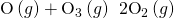### Termolecular Elementary Reactions

An elementary termolecular reaction involves the simultaneous collision of three atoms, molecules, or ions. Termolecular elementary reactions are uncommon because the probability of three particles colliding simultaneously is less than one one-thousandth of the probability of two particles colliding. There are, however, a few established termolecular elementary reactions. The reaction of nitric oxide with oxygen appears to involve termolecular steps: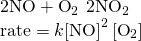Likewise, the reaction of nitric oxide with chlorine appears to involve termolecular steps: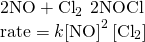### Relating Reaction Mechanisms to Rate Laws

It’s often the case that one step in a multistep reaction mechanism is significantly slower than the others. Because a reaction cannot proceed faster than its slowest step, this step will limit the rate at which the overall reaction occurs. The slowest step is therefore called the rate-limiting step (or rate-determining step) of the reaction (Figure).

A cattle chute is a nonchemical example of a rate-determining step. Cattle can only be moved from one holding pen to another as quickly as one animal can make its way through the chute. (credit: Loren Kerns)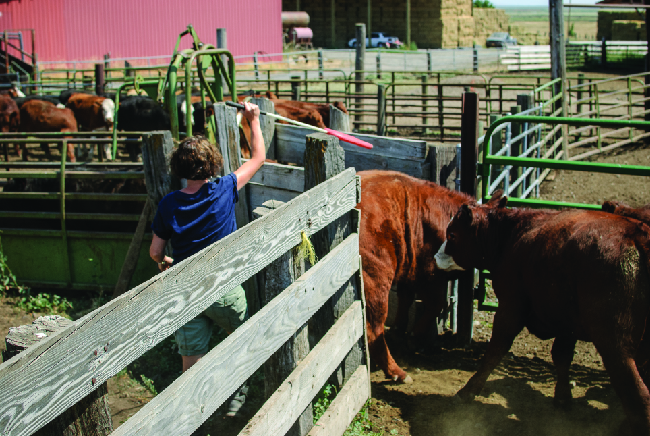As described earlier, rate laws may be derived directly from the chemical equations for elementary reactions. This is not the case, however, for ordinary chemical reactions. The balanced equations most often encountered represent the overall change for some chemical system, and very often this is the result of some multistep reaction mechanisms. In every case, the rate law must be determined from experimental data and the reaction mechanism subsequently deduced from the rate law (and sometimes from other data). The reaction of NO2 and CO provides an illustrative example: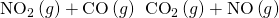For temperatures above 225 °C, the rate law has been found to be: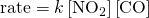The reaction is first order with respect to NO2 and first-order with respect to CO. This is consistent with a single-step bimolecular mechanism and it is possible that this is the mechanism for this reaction at high temperatures.

At temperatures below 225 °C, the reaction is described by a rate law that is second order with respect to NO2: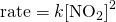This rate law is not consistent with the single-step mechanism, but is consistent with the following two-step mechanism: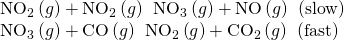The rate-determining (slower) step gives a rate law showing second-order dependence on the NO2 concentration, and the sum of the two equations gives the net overall reaction.

In general, when the rate-determining (slower) step is the first step in a mechanism, the rate law for the overall reaction is the same as the rate law for this step. However, when the rate-determining step is preceded by a step involving a rapidly reversible reaction the rate law for the overall reaction may be more difficult to derive.

As discussed in several chapters of this text, a reversible reaction is at equilibrium when the rates of the forward and reverse processes are equal. Consider the reversible elementary reaction in which NO dimerizes to yield an intermediate species N2O2. When this reaction is at equilibrium: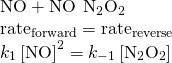This expression may be rearranged to express the concentration of the intermediate in terms of the reactant NO: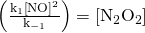Since intermediate species concentrations are not used in formulating rate laws for overall reactions, this approach is sometimes necessary, as illustrated in the following example exercise.

Deriving a Rate Law from a Reaction Mechanism The two-step mechanism below has been proposed for a reaction between nitrogen monoxide and molecular chlorine: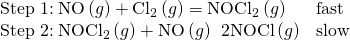Use this mechanism to derive the equation and predicted rate law for the overall reaction.

Solution The equation for the overall reaction is obtained by adding the two elementary reactions: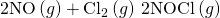To derive a rate law from this mechanism, first write rates laws for each of the two steps.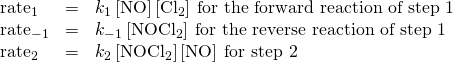Step 2 is the rate-determining step, and so the rate law for the overall reaction should be the same as for this step. However, the step 2 rate law, as written, contains an intermediate species concentration, [NOCl2]. To remedy this, use the first step’s rate laws to derive an expression for the intermediate concentration in terms of the reactant concentrations.

Assuming step 1 is at equilibrium: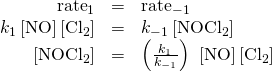Substituting this expression into the rate law for step 2 yields: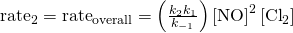Check Your Learning The first step of a proposed multistep mechanism is: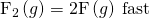Derive the equation relating atomic fluorine concentration to molecular fluorine concentration.

Answer: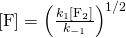### Key Concepts and Summary

The sequence of individual steps, or elementary reactions, by which reactants are converted into products during the course of a reaction is called the reaction mechanism. The molecularity of an elementary reaction is the number of reactant species involved, typically one (unimolecular), two (bimolecular), or, less commonly, three (termolecular). The overall rate of a reaction is determined by the rate of the slowest in its mechanism, called the rate-determining step. Unimolecular elementary reactions have first-order rate laws, while bimolecular elementary reactions have second-order rate laws. By comparing the rate laws derived from a reaction mechanism to that determined experimentally, the mechanism may be deemed either incorrect or plausible.

### Chemistry End of Chapter Exercises

Why are elementary reactions involving three or more reactants very uncommon?

In general, can we predict the effect of doubling the concentration of A on the rate of the overall reaction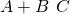? Can we predict the effect if the reaction is known to be an elementary reaction?

No. In general, for the overall reaction, we cannot predict the effect of changing the concentration without knowing the rate law. Yes. If the reaction is an elementary reaction, then doubling the concentration of A doubles the rate.

Define these terms:

(a) unimolecular reaction

(b) bimolecular reaction

(c) elementary reaction

(d) overall reaction

What is the rate law for the elementary termolecular reaction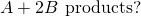For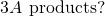Rate = k[A][B]2; Rate = k[A]3

Given the following reactions and the corresponding rate laws, in which of the reactions might the elementary reaction and the overall reaction be the same?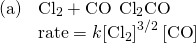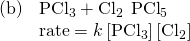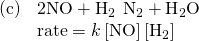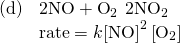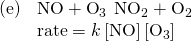Write the rate law for each of the following elementary reactions:

(a)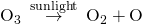(b)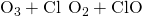(c)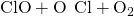(d)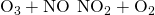(e)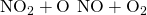(a) Rate1 = k[O3]; (b) Rate2 = k[O3][Cl]; (c) Rate3 = k[ClO][O]; (d) Rate2 = k[O3][NO]; (e) Rate3 = k[NO2][O]

Nitrogen monoxide, NO, reacts with hydrogen, H2, according to the following equation: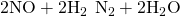What would the rate law be if the mechanism for this reaction were: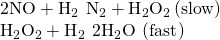Experiments were conducted to study the rate of the reaction represented by this equation.1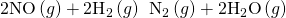Initial concentrations and rates of reaction are given here.

Experiment Initial Concentration [NO] (mol L−1) Initial Concentration, [H2] (mol L−1 min−1) Initial Rate of Formation of N2 (mol L−1 min−1)
1 0.0060 0.0010 1.810−4
2 0.0060 0.0020 3.610−4
3 0.0010 0.0060 0.3010−4
4 0.0020 0.0060 1.210−4

Consider the following questions:

(a) Determine the order for each of the reactants, NO and H2, from the data given and show your reasoning.

(b) Write the overall rate law for the reaction.

(c) Calculate the value of the rate constant, k, for the reaction. Include units.

(d) For experiment 2, calculate the concentration of NO remaining when exactly one-half of the original amount of H2 had been consumed.

(e) The following sequence of elementary steps is a proposed mechanism for the reaction.

Step 1: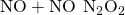Step 2: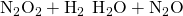Step 3: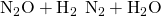Based on the data presented, which of these is the rate determining step? Show that the mechanism is consistent with the observed rate law for the reaction and the overall stoichiometry of the reaction.

(a) Doubling [H2] doubles the rate. [H2] must enter the rate law to the first power. Doubling [NO] increases the rate by a factor of 4. [NO] must enter the rate law to the second power. (b) Rate = k [NO]2[H2]; (c) k = 5.0103 mol−2 L−2 min−1; (d) 0.0050 mol/L; (e) Step II is the rate-determining step. If step I gives N2O2 in adequate amount, steps 1 and 2 combine to give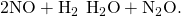This reaction corresponds to the observed rate law. Combine steps 1 and 2 with step 3, which occurs by supposition in a rapid fashion, to give the appropriate stoichiometry.

The reaction of CO with Cl2 gives phosgene (COCl2), a nerve gas that was used in World War I. Use the mechanism shown here to complete the following exercises: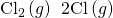(fast, k1 represents the forward rate constant, k−1 the reverse rate constant)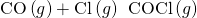(slow, k2 the rate constant)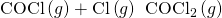(fast, k3 the rate constant)

(a) Write the overall reaction.

(b) Identify all intermediates.

(c) Write the rate law for each elementary reaction.

(d) Write the overall rate law expression.

### Footnotes

• 1This question is taken from the Chemistry Advanced Placement Examination and is used with the permission of the Educational Testing Service.

### Glossary

bimolecular reaction
elementary reaction involving two reactant species
elementary reaction
reaction that takes place in a single step, precisely as depicted in its chemical equation
intermediate
species produced in one step of a reaction mechanism and consumed in a subsequent step
molecularity
number of reactant species involved in an elementary reaction
rate-determining step
(also, rate-limiting step) slowest elementary reaction in a reaction mechanism; determines the rate of the overall reaction
reaction mechanism
stepwise sequence of elementary reactions by which a chemical change takes place
termolecular reaction
elementary reaction involving three reactant species
unimolecular reaction
elementary reaction involving a single reactant species

## LicenseChemistry 2e by OSCRiceUniversity is licensed under a Creative Commons Attribution 4.0 International License, except where otherwise noted.